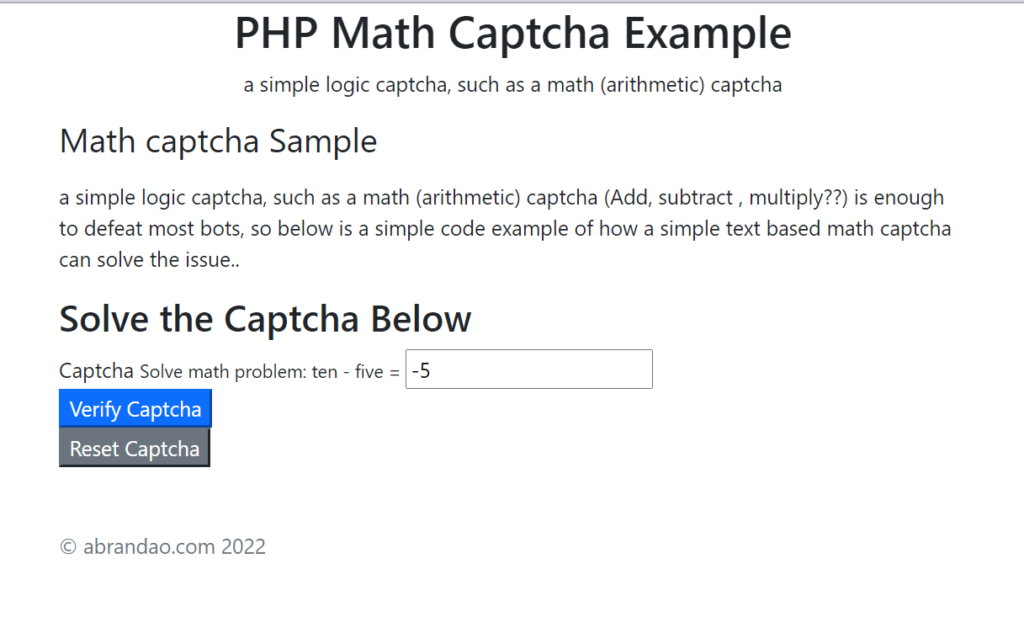# Simple PHP math logic captcha – to help prevent form spam10

## Why a simple PHP Math Captcha ?

If you’re reading this you’re probably aware there are a ton of on-line captcha services like Re-Captcha, hCaptcha ,to help keep your forms and user submitted input free of bot spam,  and other nonsense.  But most require registration with capthca service, setting up API keys etc, so it’s a fair bit amount of work to configure it , and integrate it with your code, especially if you have a low volume , not terribly sensitive form, to make it worth the effort.

But more often than not a simple logic captcha, such as a  math (arithmetic) captcha (Add, subtract , multiply??) is enough to defeat most bots, so below is a simple code example of how a simple text based math captcha can solve the issue.. Demo and Github code below..

Let’s begin with the basics we’ll create a simple arithmetic math problem like 5+3 then ask the user to supply and answer, then we’ll capture the user’s input and compare the result with the correct solution on the form before we do anything with the input. Since most bots may not (some of the more sophisticated ones do recognition, but most do not), they’ll just fail and NOT send you the form/ or capture the user input.

Here’s the really basic code:

Essentially it sets up a couple of arrays:

• one of the operations like +, – , *  \$ops
• one of the digits 1-10 as both numbers and words \$numbers
• It then randomly chooses the first and second numbers from a range of 0..10
• We then use those values as the indexes into the corresponding arrays \$numbers  and \$ops
• We then put the regular \$n1 \$op \$n2 into an eval function to get our answer as \$computed
• For encoding the actual answer we use and MD5 hash to  create a one-way hash , we’ll compare this hash later with the user supplied value,
```<?php
\$ops=["+","-","*"];
\$numbers=["0","one","2","three","4","five","6","seven","8","nine","ten"];
\$n1= rand(0,10);
\$n2= rand(0,10);
\$op=\$ops[rand(0,2)];
echo \$numbers[\$n1]." ".\$op." ".\$numbers[\$n2]." = ";
\$computed= eval('return '.\$n2.\$op.\$n1.';');
\$val=md5(\$computed);
// echo "<input type='hidden' name='answer' value='\$computed'>"; //debugging purposes

?>
```

and the form would appear something like this.you would then provide the proper input tag to capture the users input like, because this Captcha uses a mix of numbers and worked numbers, you may want to add clarity to the user that all the answers need to be as numbers. Here’s the code portion of the Form.

```<form action="captcha_check.php" method="POST">
<h2> Solve the Captcha Below </h2>
<div class="field">

<small> Solve  math problem:
<!-- PHP Math Captcha Code goes here --->
<?php
\$ops=["+","-","*"];
\$numbers=["0","won","2","three","4","five","6","seven","8","nine","ten"];
\$n1= rand(0,10);
\$n2= rand(0,10);
\$op=\$ops[rand(0,2)];
echo \$numbers[\$n1]." ".\$op." ".\$numbers[\$n2]." = ";
\$computed= eval('return '.\$n2.\$op.\$n1.';');
\$val=md5(\$computed);
// more secure way is to add a salt value to the \$computed number to prevent md5 brute-force
// \$salt_value=12345;
// \$val=md5(\$computed+\$salt_value); //\$salt_value can be any number
// echo "<input type='hidden' name='answer' value='\$computed'>"; //debugging purposes
?>
</small>
<input type="text" name="captchauser" id="captchauser" required />  <!-- this is the User Input we Use -->
</div>

</form>```

Finally on the form where you are going to collect the data you would verify the Math captcha is correct.

```<?php

try {

// Do something here with your input

throw new Exception("Captcha answer is not correct. No message sent. <a href='index.php#contact' >Re-try</a> ");

// Continue.. with the normal flow of capturing data input

} catch (Exception \$e) {
echo "Message could not be sent. Error:".\$e->getMessage() ;
}

?>
```

That’s it very simple , and pretty effective against defeating most Spambots. You can of course increase the complexity of this captcha using things like more than just on \$operation , or add more numbers, or mispell  the numbers or add more operations etc..

The point is it becomes exponentially harder for the bots to determine the answer, while still keeping the code clean, simple and straightforward without a lot external dependencies. My favorite type of code..

## 10 thoughts on “Simple PHP math logic captcha – to help prevent form spam”

1.Reply Json

thanks that looks great.

2.Reply Bryce Williams

How can you make this do a quiz like ask what color is blood?

3.Reply mathieu

Hi, great solution, but I’m stuck.

Could you please publish the html/php source of your Sample form to understand how to call/insert the problem to solve?

mathieu

•Reply

Mathieu,
I updated the page and added a working demo..
Find the working demo of Math Captcha Demonstration here
Find source code to this demo here on GithubGithub Match Captcha

If this helps let me know and feel free to share the link.

🙂 , there’s no captcha on this blog comments, since WP does a pretty good job of filtering out spam comments. so they did the hard work already.

4.Reply Jane8295

Works great , but many of the bots I’ve seen already are good at doing simple math problems… What can we do to make it more difficult?

5.Reply mathieu

Hi Tony,

Thanks for having updated this tutorial.

For security reason, I won’t choose WP but Hugo CMS a static site generator.

That’s why I need a CAPTCHA!

Mathieu

6.Reply mathieu

Hi tony,

There’s a bug, it doesn’t work when the result is negative number.

Example:

‘won – ten’ = -9

The captcha fails character ‘-‘ is not a number?

•Reply Anonymous

Thanks, will update..

7.Reply Bony

•Reply